# Comments requested on MOSFET high-side driver

Introduction

I find I learn a lot by writing tutorials, because when you write a tutorial you want to explain exactly why you did something, rather than something else. Also it helps you clarify concepts in your mind (my mind, that is).

I am working on a page about interfacing with a high-side driver (ie. to source current rather than sink it) using a P-channel MOSFET.

There are some great YouTube videos about this subject, but I find at times that I want to just "glance at a circuit" rather than sit there watching a video for 10 minutes, to try to find the part where they explain about the relationship between current, RDSOn and heat.

Schematic

Below is the proposed schematic: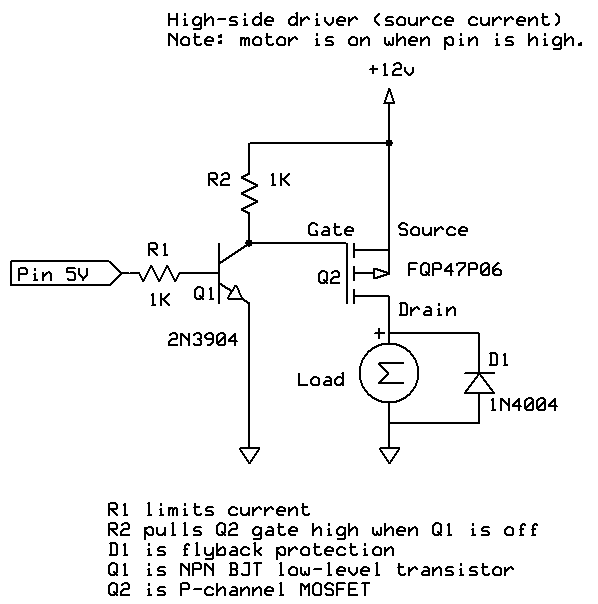For testing, the MOSFET is a FQP47P06 and the transistor is a 2N3904.

You are welcome to make suggestions about the values of the components (eg. R1 and R2).

Also D1, I am using a 1N4148 for a 300 mA motor. Would this be adequate? Would a Schottky be better, or is that overkill? I believe the 1N4148 is a fast (4nS) recovery, 200 mA, 100V diode.

I have tested with a 2 ohm load, driving the whole thing from a power supply limited to 1 amp. The input pin is a 1 kHz square wave (at 5V).

Overall results are: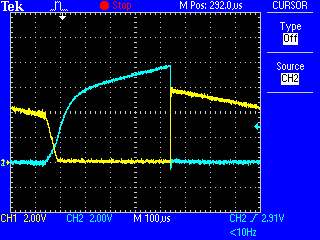The blue trace is the Gate of Q2 and the yellow trace is the Drain of Q2.

As expected, the FET turns on when the gate becomes more negative, and turns off when the gate becomes less negative. In particular: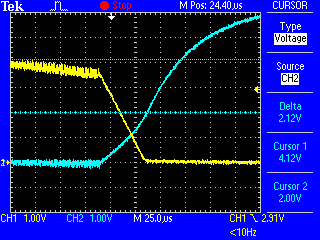The delta there is around 2V which agrees with the datasheet which quotes VGS(th) as -2V mininum.

Turn-off time

Once concern I have is the fairly lengthy turn-off time: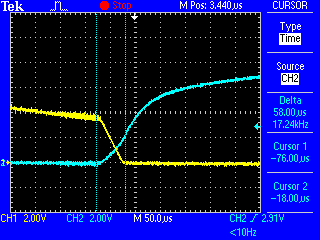That is around 58 µS which seems a lot. It also accounts for, I think, that the FET is getting hot (around 30 °C) because it is running unsaturated for quite a while.

Would a lower value for R2 help here? Any suggested values? If R2 was lower, then R1 might need to be lower too to provide adequate current to sink the gate to 0V when required. Would that be right?

Turn-on time

Turn-on time seems to be somewhat faster (around 1 µS) which seems OK to me: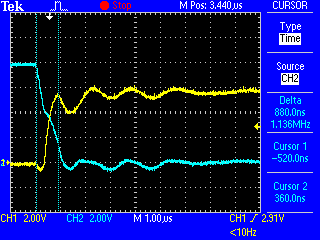Power consumption and heat

I calculate the power through the load as being I2R where I is 1 and RDS(on) is 0.021, thus:

``````power = 1 * 1 * 0.021 = 0.021 watts
``````

The "Thermal Resistance Junction to Ambient" (RØJA) is 62.5 °C/W, thus I expect a rise of:

``````0.021 * 62.5 = 1.3125  °C  (over ambient)
``````

Does that sound right? However I am measuring more like 10 degrees rise.

Any other comments or suggestions?

Yes, a lower R2. 10k is a pretty high resistance to discharge the gate charge.

Unless you are on a tight current budget from the Arduino pins, I think I'd start with a 1k resistor for R1, and 470 ohms for R2.

Using a 200mA rated diode on a 300mA motor seems unwise. I'd use at least a 1A diode. As has already been discussed, a 1N400X diode is fast enough turn-on time, it is the turn-off time that is slow.

Come to think of it, I think the description of R2 on the schematic ("R2 is pull-up") is wrong. More precise wording is solicited. :)

Hm. Well, it -is- a pull-up in the sense that we consider positive voltage on a schematic to be "up".

Its job is to discharge the charge built up on the Gate-Source "capacitor". It is -not- there in case of high impedance. It is there so that when Q1 is turned OFF, the gate is discharged and Q2 turns off. Otherwise it will stay on for a long time, perhaps never turning off once it is turned on due to Q1 leakage.

Or if not Q1 not turned on, Q2 may eventually start drifting into conduction due to Q2 gate charging from Q1 leakage current.

I would make R2 smaller, say 1K to reduce the turn off time of the FET.

I would also use a 1N4001 diode, that one is just a signal diode.

Cheers

I think I'd start with a 1k resistor for R1, and 470 ohms for R2.

So to do the maths:

1k resistor for R1 and assuming a gain of 100 (actually HFE = 300 on the datasheet) would give:

``````(5 - 0.7) / 1000 * 100 = 0.43  (430 mA)
``````

So Q1 can sink 430 mA.

470 ohms for R2, means to drop 12V:

``````12 / 470 = 0.026  (26 mA)
``````

Power through R2:

``````0.026^2 * 470 = 0.318  (318 mW)
``````

Thermal resistance of Q1 (RØJA = 200):

`````` 0.318 * 200 = 63.6 °C
``````

That seems a bit hot, or did I get my calculations wrong?

Incorrect. You never use the linear small signal beta to calculate saturation current.

Use more like 10 or 20. For a 2N3904, the datasheet says 10x.

Page 3, it rates saturation VCE for IC = 10mA when IB = 1mA, and IC = 50mA when IB = 5mA. http://www.physics.csbsju.edu/~awhitten/phys358/reference/2N3904_4.pdf

So for 4.3mA, don't count on more than 43mA IC through Q2.

You should always get a resistor with a power rating at least 2x the power that will be dissipated.

It looks like you just took the power in R2 and used it to calculate heat in Q1.

Q1 power is the sum of power from the base and the collector currents and drops: 0.95 * 0.0043 + 0.2 * 0.026 = 9.285mW

In fact, from the datasheet 200mV drop VCE is maximum. It is usually less than that. But plan for the maximum.

I would have said "Power -in- R2".

OK just to make sure:

Q1 power is the sum of power from the base and the collector currents and drops: 0.95 * 0.0043 + 0.2 * 0.026 = 9.285mW

VBE(sat) = 0.95 5V (at pin) - VBE(sat) / 1k = (5 - 0.95) / 1000 = 0.00405 (4.05 mA) VCE (sat) = 0.2 26 mA to sink through the resistor

Thus:

Power = 0.95 * 0.00405 + 0.2 * 0.026 = 0.0090475 (9.05 mW)

Heat gain = 0.0090475 * 200 = 1.81 °C

Does that sound right?

Whoops, forgot to correct the base current with the higher VBE. :-[

That looks right.

Changing R2 to 470 ohms seems to have reduced the rise time at the gate to 5.5 µS.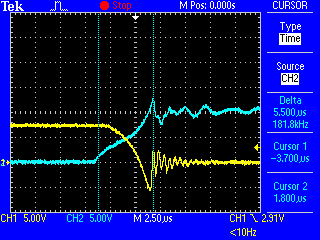From 58uS to 5.5uS. Sounds about right.

One of the problems you run into with a MOSFET is drain to gate capacitance. So as the gate is discharging (going high), the drain is going low, and the capacitance between gate and drain slows the discharge of the gate by providing some charging current.

That is probably part of why a 20:1 change in resistance only resulted in an 11:1 change in Off switching time.

So it would be reasonable to suggest, other things being equal, that if we are doing PWM, to reduce the frequency, so that these "edge cases" happen less often? Obviously this problem goes away if we are switching infrequently, like turning garden lights on and off.

I have finished the tentative tutorial, which is at http://www.gammon.com.au/forum/?id=12737.

There is more explanation in the tutorial than I had above, plus a low-side driver (to sink current). If you spot any mistakes please let me know.

@polymorph - I scaled R2 back to 1k, it seemed we were getting diminishing returns on low-value resistors, and that would keep the current drain on Q1 down. The switching time is now about 7.5 µS.

How is the MOSFET temperature with the new resistors?

I use MOVs when switching transformers and solenoids and believe you could get much greater speed improvement with your MOSFET high-side motor driver. The speed improvement comes from clamping higher than the 0.7V of a diode. I haven't used MOVs across a motor, but I wonder what speed improvements could be had with an MOV rated for 16V DC such as this: http://www.digikey.com/product-detail/en/B72210S1140K551/495-6514-1-ND/4931646

Also check this post: http://forum.arduino.cc/index.php?topic=294268.msg2064128#msg2064128

Good trade-off.

Yes, if the application is fine with a slower PWM, that saves switching losses and the other losses associated with it like driver current losses.

The 1N4001 is not a fast switching diode. It is a garden variety line frequency rectifier, but it switches On fast enough to absorb most inductive transients. What dlloyd is referring to is more of an issue with the speed of magnetic field collapse in a coil. This is an issue with relays as a low clamp voltage can cause contact burning as it slows release. In that case, adding a resistor in series with the clamping diode can speed that up.

For a motor, it is going to generate a voltage as it continues to turn, but it will be in the same polarity as the voltage applied and not spike up any higher. Well, except a motor is also made of inductors, so I'd still keep the diode.

For your MOSFET power calculations, I'd say 2^2 rather than 4. It is clearer, then, that the number is 2A squared.

I try, as a general rule, to keep device temperatures under 75C when possible. 150C may be a maximum, but by mentioning, you may be unintentionally giving people the impression that it doesn't really have any drawbacks.

[quote author=Coding Badly link=msg=2066891 date=1422591565] How is the MOSFET temperature with the new resistors? [/quote]

Well, it still got up to around 32 °C, which I am not that impressed with, but I think my test conditions are not helping. With a fixed current from the lab supply it is dropping the voltage to around 3.6V which then means I think it is not properly switching on.

I think I'll retry with a lower current, thus allowing the voltage to rise.

For your MOSFET power calculations, I'd say 2^2 rather than 4. It is clearer, then, that the number is 2A squared.

Fixed.

Ach, make that a higher current, allowing the voltage to rise. But then the higher current heats the thing up more.

It’s tricky setting up good test conditions.